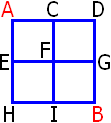## Tuesday, January 5, 2010

### Solution to two math problems

See the original problems

Putnam problem A1:

Take any two points, call them A and B. Construct four squares as shown below:For each of the four squares, you can write out an equation. You can also write out an equation for the larger square ADBH. Combining all these, you can show that f(A) + f(B) = 0

So if there were any point P such that f(P) = c, then the function f would be -c on the rest of the plane. This doesn't work unless c = 0. Therefore f is zero on the entire plane.

Coin-flipping game:

This is the formula for the expected N, given that N is odd:

(1/2 + 3/8 + 5/32 + 7/128 + ...) / (1/2 + 1/8 + 1/32 + 1/128 + ...)

Those infinite sums actually aren't too hard to calculate if you know what you're doing. It comes out to 5/3, so a fair price would be \$1.67.

But if you are really averse to calculating infinite sums, there are other ways to do it. You can write a computer simulation, for instance. Some puzzle purists think that's sort of cheating, but I think it's very practical. I wrote one myself to check my answer, and what do you know, I had made a miscalculation on my first try.

I have another clever solution, but it's tough to follow.
First we need to know the probability that N is odd in any given attempt of the game. Let's call this value P(O).

P(O) = (1/2 + 1/8 + 1/32 + ...)
= (1/2 + 1/8 + 1/32 + ...) / 1
= (1/2 + 1/8 + 1/32 + ...) / (1/2 + 1/4 + 1/8 + 1/16 + 1/32 + 1/64 + ...)
= (2/4 + 2/16 + 2/64 + ...) / (3/4 + 3/16 + 3/64 + ...)
P(O) = 2/3

Let's also name a few other numbers:
P(E) = probability that N is even = 1/3
E(N) = expected value of N = 2
E(N|O) = expected value of N, given that N is odd
E(N|E) = expected value of N, given that N is even

We also use the identity:
E(N) = E(N|O) * P(O) + E(N|E) * P(E)

We also need a key realization. If you ignore the first coin, E(N|E) is exactly the same as E(N|O). So we have the following equation:
E(N|E) = E(N|O) + 1

Combining these two equations, and the known values, we can calculate:
E(N|O) = 5/3

So 5/3, or \$1.67, is our answer.
This problem may seem all abstract, but I was thinking about it because it came up in a physics discussion. I was arguing with one of my professors about muon decay rates. But that's too complicated, so I won't go into it.

Yeah, so, I realize some of these solutions are kinda hard to understand. Just ask! For instance, I can go ahead and explain how to calculate that infinite sum if people are really interested.